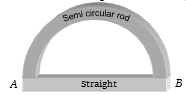The temperature of a liquid drops from 365 K to 361 K in 2 minutes. Find the time during which temperature of the liquid drops from 344 K to 342 K . Temperature of room is 293 K
(a) 84 sec                        (b) 72 sec
(c) 66 sec                        (d) 60 sec

Concept Questions :-

Newton's law of cooling
High Yielding Test Series + Question Bank - NEET 2020

Difficulty Level:

Newton’s law of cooling, holds good only if the temperature difference between the body and the surroundings is
(a) Less than 10$°\mathrm{C}$       (b) More than 10$°\mathrm{C}$
(c) Less than 100$°\mathrm{C}$      (d) More than 100$°\mathrm{C}$

Concept Questions :-

Newton's law of cooling

Difficulty Level:

The temperature of a body falls from 50$°\mathrm{C}$ to 40$°\mathrm{C}$ in 10 minutes. If the temperature of the surroundings is 20$°\mathrm{C}$ Then temperature of the body after another 10 minutes will be
(a) 36.6$°\mathrm{C}$            (b) 33.3$°\mathrm{C}$
(c) 35$°\mathrm{C}$               (d) 30$°\mathrm{C}$

Concept Questions :-

Newton's law of cooling
High Yielding Test Series + Question Bank - NEET 2020

Difficulty Level:

A body takes 5 minutes to cool from 90$°\mathrm{C}$ to 60$°\mathrm{C}$. If the temperature of the surroundings is 20$°\mathrm{C}$, the time taken by it to cool from 60$°\mathrm{C}$ to 30$°\mathrm{C}$ will be.
(a) 5 min                    (b) 8 min
(c) 11 min                   (d) 12 min

Concept Questions :-

Newton's law of cooling
High Yielding Test Series + Question Bank - NEET 2020

Difficulty Level:

An object is cooled from 75°C to 65°C in 2 minutes in a room at 30°C. The time taken to cool another object from 55°C to 45°C in the same room in minutes is -

(a) 4                   (b) 5
(c) 6                   (d) 7

Concept Questions :-

Newton's law of cooling
High Yielding Test Series + Question Bank - NEET 2020

Difficulty Level:

A cane is taken out from a refrigerator at 0°C. The atmospheric temperature is 25°C. If ${\mathrm{t}}_{1}$ is the time taken to heat from 0°C to 5°C and ${\mathrm{t}}_{2}$ is the time taken from 10°C to 15°C, then
(a) ${\mathrm{t}}_{1}>{\mathrm{t}}_{2}$                         (b) ${\mathrm{t}}_{1}<{\mathrm{t}}_{2}$
(c) ${\mathrm{t}}_{1}={\mathrm{t}}_{2}$                         (d) There is no relation

Concept Questions :-

Newton's law of cooling
High Yielding Test Series + Question Bank - NEET 2020

Difficulty Level:

Two rods (one semi-circular and other straight) of same material and of same cross-sectional area are joined as shown in the figure. The points A and B are maintained at different temperature. The ratio of the heat transferred through a cross-section of a semi-circular rod to the heat transferred through a cross section of the straight rod in a given time is(a) 2 : $\mathrm{\pi }$
(b) 1 : 2
(c) $\mathrm{\pi }$ : 2
(d) 3 : 2

Concept Questions :-

Conduction
High Yielding Test Series + Question Bank - NEET 2020

Difficulty Level:

A wall is made up of two layers A and B. The thickness of the two layers is the same, but materials are different. The thermal conductivity of A is double than that of B. In thermal equilibrium the temperature difference between the two ends is 36$°\mathrm{C}$. Then the difference of temperature at the two surfaces of A will be

(a) 6$°\mathrm{C}$             (b) 12$°\mathrm{C}$
(c) 18$°\mathrm{C}$            (d) 24$°\mathrm{C}$

Concept Questions :-

Conduction
High Yielding Test Series + Question Bank - NEET 2020

Difficulty Level:

Ice starts forming in lake with water at 0$°\mathrm{C}$ and when the atmospheric temperature is -10$°\mathrm{C}$. If the time taken for 1 cm of ice be 7 hours, then the time taken for the thickness of ice to change from 1 cm to 2 cm is

(a) 7 hours                                   (b) 14 hours
(c) Less than 7 hours                     (d) More than 7 hours

Concept Questions :-

Conduction
High Yielding Test Series + Question Bank - NEET 2020

Difficulty Level:

A cylinder of radius R made of a material of thermal conductivity ${\mathrm{K}}_{1}$ is surrounded by a cylindrical shell of inner radius R and outer radius 2R made of material of thermal conductivity ${\mathrm{K}}_{2}$. The two ends of the combined system are maintained at two different temperatures. There is no loss of heat across the cylindrical surface and the system is in steady state. The effective thermal conductivity of the system is
(a) ${\mathrm{K}}_{1}+{\mathrm{K}}_{2}$           (b) $\frac{{\mathrm{K}}_{1}{\mathrm{K}}_{2}}{{\mathrm{K}}_{1}+{\mathrm{K}}_{2}}$
(c) $\frac{{\mathrm{K}}_{1}+3{\mathrm{K}}_{2}}{4}$        (d) $\frac{3{\mathrm{K}}_{1}+{\mathrm{K}}_{2}}{4}$

Concept Questions :-

Conduction